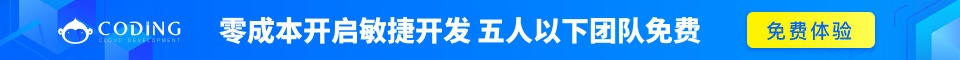# Python 类

Python中的类提供了面向对象编程的所有基本功能：类的继承机制允许多个基类，派生类可以覆盖基类中的任何方法，方法中可以调用基类中的同名方法。

## 类定义

```class ClassName:
<statement-1>
.
.
.
<statement-N>
```

## 类对象

```class MyClass:
"""A simple example class"""
i = 12345
def f(self):
return 'hello world'
```

```x = MyClass()
```

```def __init__(self):
self.data = []
```

```x = MyClass()
```

```>>> class Complex:
...     def __init__(self, realpart, imagpart):
...         self.r = realpart
...         self.i = imagpart
...
>>> x = Complex(3.0, -4.5)
>>> x.r, x.i
(3.0, -4.5)
```

## 类的方法

```#类定义
class people:
#定义基本属性
name = ''
age = 0
#定义私有属性,私有属性在类外部无法直接进行访问
__weight = 0
#定义构造方法
def __init__(self,n,a,w):
self.name = n
self.age = a
self.__weight = w
def speak(self):
print("%s is speaking: I am %d years old" %(self.name,self.age))

p = people('tom',10,30)
p.speak()
```

## 继承

Python 同样支持类的继承，如果一种语言不支持继承就，类就没有什么意义。派生类的定义如下所示:

```class DerivedClassName(BaseClassName1):
<statement-1>
.
.
.
<statement-N>
```

BaseClassName（示例中的基类名）必须与派生类定义在一个作用域内。除了类，还可以用表达式，基类定义在另一个模块中时这一点非常有用:

```class DerivedClassName(modname.BaseClassName):
```

### 实例

```#单继承示例
class student(people):
def __init__(self,n,a,w,g):
#调用父类的构函
people.__init__(self,n,a,w)
#覆写父类的方法
def speak(self):
print("%s is speaking: I am %d years old,and I am in grade %d"%(self.name,self.age,self.grade))

s = student('ken',20,60,3)
s.speak()
```

## 多重继承

Python同样有限的支持多继承形式。多继承的类定义形如下例:

```class DerivedClassName(Base1, Base2, Base3):
<statement-1>
.
.
.
<statement-N>
```

```#另一个类，多重继承之前的准备
class speaker():
topic = ''
name = ''
def __init__(self,n,t):
self.name = n
self.topic = t
def speak(self):
print("I am %s,I am a speaker!My topic is %s"%(self.name,self.topic))

#多重继承
class sample(speaker,student):
a =''
def __init__(self,n,a,w,g,t):
student.__init__(self,n,a,w,g)
speaker.__init__(self,n,t)

test = sample("Tim",25,80,4,"Python")
test.speak()#方法名同，默认调用的是在括号中排前地父类的方法
```

## 类私有方法

__private_method 两个下划线开头，声明该方法为私有方法，不能在类地外部调用。

• __init__ 构造函数，在生成对象时调用
• __del__ 析构函数，释放对象时使用
• __repr__ 打印，转换
• __setitem__按照索引赋值
• __getitem__按照索引获取值
• __len__获得长度
• __cmp__比较运算
• __call__函数调用